﻿ Moment Problem and Inverse Cauchy Problems for Heat Equation

### Moment Problem and Inverse Cauchy Problems for Heat Equation

O. Yaremko, N. Yaremko, T. EliseevaOPEN ACCESSPEER-REVIEWED

## Moment Problem and Inverse Cauchy Problems for Heat Equation

O. Yaremko1, N. Yaremko1,, T. Eliseeva1

1Penza State University, Penza, Russia

### Abstract

The solution of Hamburger and Stieltjes moment problem can be thought of as the solution of a certain inverse Cauchy problem. The solution of the inverse Cauchy problem for heat equation is founded in the form of Hermite polynomial series. The author reveals, the formulas obtained by him for the solution of inverse Cauchy problem have a symmetry with respect to the formulas for corresponding direct Cauchy problem. Obtained formulas for solution of the inverse problems can serve as a basis for the solution of the moment problem.

• Yaremko, O., N. Yaremko, and T. Eliseeva. "Moment Problem and Inverse Cauchy Problems for Heat Equation." Turkish Journal of Analysis and Number Theory 3.1 (2015): 33-36.
• Yaremko, O. , Yaremko, N. , & Eliseeva, T. (2015). Moment Problem and Inverse Cauchy Problems for Heat Equation. Turkish Journal of Analysis and Number Theory, 3(1), 33-36.
• Yaremko, O., N. Yaremko, and T. Eliseeva. "Moment Problem and Inverse Cauchy Problems for Heat Equation." Turkish Journal of Analysis and Number Theory 3, no. 1 (2015): 33-36.

 Import into BibTeX Import into EndNote Import into RefMan Import into RefWorks

### 1. Introduction

Motivated by considerations arising from many-body quantum physics the authors consider the moment problem in the general case where the moments are finite real numbers. They present a well- defined analytic procedure for the construction of an infinite set of exact solutions to the above problem and discuss several special cases. Before the development of Quantum Mechanics, mathematicians were interested in understanding the relationship between a positive function,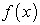and its (power) moments,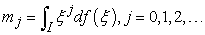(1)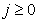and integer. The basic question was, what properties must all of the moments have in order to conclude that the underlying function is positive, or nonnegative (i.e.,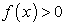This is referred to as the Moment Problem [1, 7, 8]. Depending on the nature of the domain,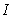, on which the function is defined, the corresponding Moment Problem is given different names. Thus, ifthe entire real axis, we call this the Hamburger moment problem, in recognition of the mathematician Hamburger who proved a particular theorem, quoted below. If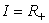, the nonnegative real axis (including zero), then we refer to the Stieltjes Moment Problem, in recognition of Stieltjes. This particular form of the Moment Problem is very important because it is related to the theory of Pade approximants . If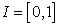, the closed unit interval, then we say that we have a Hausdorff Moment Problem. Finally, if one deals with periodic functions,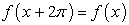(2)

then one has the Trigonometric moment problem, in which the moments are defined in terms of periodic functions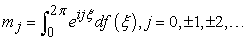(3)

Another issue is, suppose one is given the infinite set of numbers,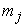, when are these the moments of a unique positive function? That is, when can we guarantee that there are no other positive functions having the same moments? This is an important concern, and leads to the Carlemann conditions for uniqueness . Although the Stieltjes moment problem was solved in the 1800s. In early 1900's, Markov  obtained significant results for both the feasibility and the bounding moment problems. Later, in 1920, Hamburger  extended the Stieltjes moment problem to the real axis, and established the moment problem as a theory of its own. In the same time, Hausdorff , defined the Hausdorff moment problem on a finite interval in connection with convergence-preserving matrices; this new approach for the moment problem was the first one not related to continued fractions. Two years later, in 1922, Nevanlinna  extended the Hamburger moment problem to the complex functions. Riesz in 1923 , was the first who extended the moment problem in functional analysis, by observing the connection between the moment problem and the space of bounded linear functional on C([a,b]).

The Hamburger moment problem is solvable (that is,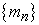is a sequence of moments) if and only if the corresponding Hankel kernel on the nonnegative integers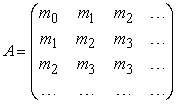is positive definite, i.e.,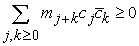for an arbitrary sequence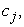of complex numbers with finite support (i.e.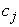except for finitely many values of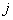).

In this paper a moment problem reduces to the inverse Cauchy problems for the heat equation. The inverse Cauchy problem for the heat equation consists of to reconstruct a priori unknown initial condition of the dynamic system from its known final condition.

The inverse Cauchy problem solution is found in the Hermite polynomial series form. We noticed the solution of inverse Cauchy problem possess the symmetry with respect to solution of the direct Cauchy problem.

The principal result of the article is the solution of the moment problem regarded as the solution of a certain inverse Cauchy problem.

### 2. The Inverse Cauchy Problem for the Heat Equation on the Real Axis

The inverse problem for the heat equation of an infinite bar is to find the unknown initial distributionof thermal field by the known thermal field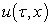([1, 2, 5, 8, 9]). This problem leads to the solving of first - type Fredholm integral equation: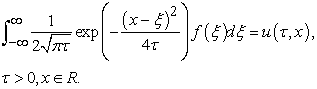(4)

The left side of equation (10) is the Poisson’s integral, . As it is shown in  the solution of equation (10) is: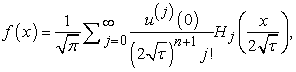(5)

where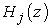Hermite polynomials :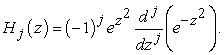(6)

We get a solution of equation (4) by the Fourier transform integral method from [10, 11]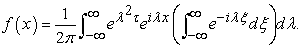If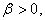then the last formula takes the form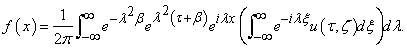(7)

The function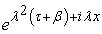is a generating function for the Hermite polynomials, this means that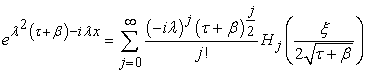(8)

Because of formula (8) we get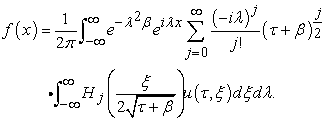We will change the order of integration and calculate the inner integral with respect to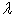. The Poisson integral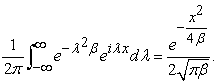is used in this calculations.

We calculate the value of an integral by- time differentiating with respect to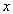: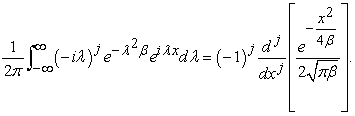On the basis of (6) we can write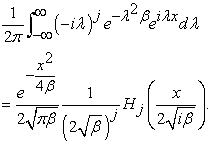Finally, first new formula for the initial distribution of the thermal field takes the form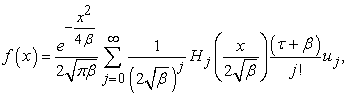(9)

where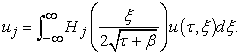### 3. Hamburger Moment Problem

Let number sequence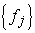defined by the formula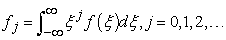then the equality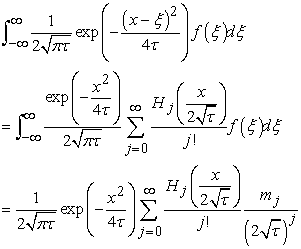is fulfilled. Let us denote function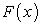by equality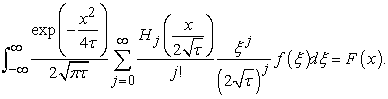The inverse problem for the heat equation of an infinite bar is consider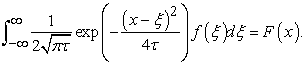Because of formula (9) we get solution of the Hamburger truncated moment problem of the form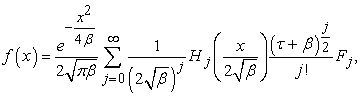(10)

where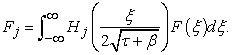### 4. The Inverse Cauchy Problem for the Heat Equation on the Nonnegative Real Axis

The inverse problem for the heat equation of an half infinite bar leads to the solving of first - type Fredholm integral equation: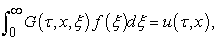(11)

where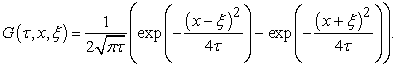The left side of equation (11) is the Poisson’s integral, . We get a solution of equation (11) by the sin- transform integral method [11, 12]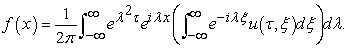Ifthen the last formula takes the form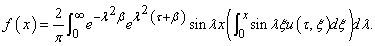(12)

The function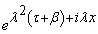is a generating function for the Hermite polynomials, this means that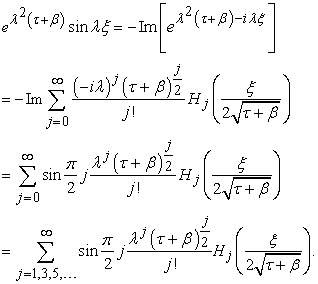(13)

Because of formula (12) we get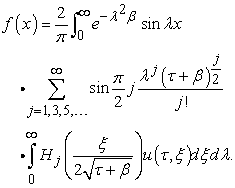We will change the order of integration and calculate the inner integral with respect to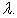The Poisson integral is used in this calculations. We getWe calculate the value of an integral by- time differentiating with respect to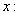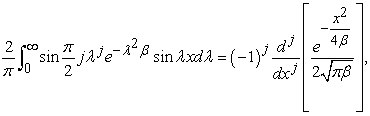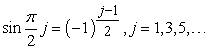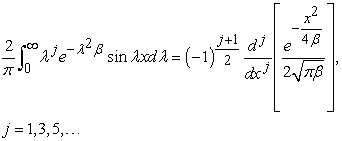On the basis of (6) we can write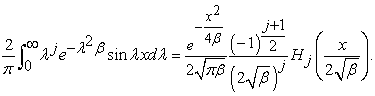Finally, first new formula for the initial distribution of the thermal field takes the form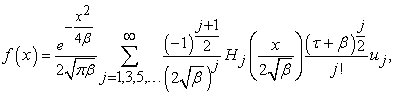where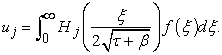### 5. Stieltjes Moment Problem

Let number sequencedefined by the formula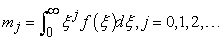then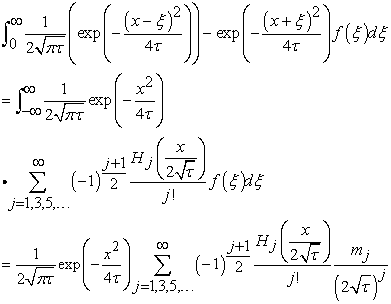let us denote this function by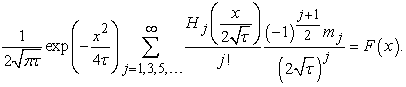We will consider the inverse problem for the heat equation of an infinite bar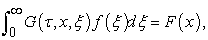where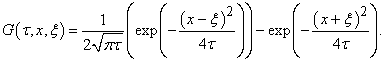Because of formula (14) we get solution of the Hamburger truncated moment problem of the form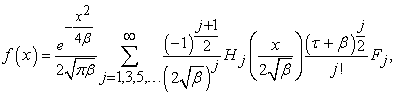where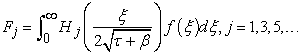### References

  Akhiezer, N.I., Krein, M.G. Some Questions in the Theory of Moments, Amer. Math. Soc., 1962.In article  Alifanov, O.M., Inverse problems of heat exchange, M, 1988, p. 279.In article  Arfken, G. B.; Weber, H. J. (2000), Mathematical Methods for Physicists (5th ed.), Boston, MA: Academic Press.In article PubMed  Bavrin, I. I., Yaremko, O. E. Transformation Operators and Boundary Value Problems in the Theory of Harmonic and Biharmonic Functions (2003) Doklady Mathematics, 68 (3), pp. 371-375.In article  Baker, G. A., Jr.; and Graves-Morris, P. Padé Approximants. Cambridge U.P., 1996.In article CrossRef  Beck, J.V., Blackwell, V., Clair, C.R., Inverse Heat Conduction. Ill-Posed Problems, M, 1989, p. 312.In article  Chebysev, P. Sur les valeurs limites des intégrales, Journal de Mathématiques pures et appliquées, 19 ( 1874), 157-160.In article  Krein, M.G. and Nudelman, A.A. The Markov Moment Problem and Extermal Problems, Translations of Mathematical Monographs, Volume Fifty, Library of Congress Cataloging in Publication Data, 1977.In article PubMed  Lavrentev, M.M., Some ill-posed problems of mathematical physics, Novosibirsk, AN SSSR, 1962, p. 92.In article  Mors, F.M., Fishbah, G. Methods of theoretical physics, 1958.In article  Yaremko, O.E. Matrix integral Fourier transforms for problems with discontinuous coefficients and transformation operators (2007) Doklady Mathematics, 76 (12), pp. 323-325.In article  Yaremko, O.E. Transformation operator and boundary value problems Differential Equation. Vol.40, No. 8, 2004, pp.1149-1160.In article CrossRef